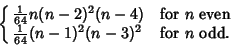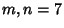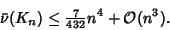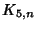## Crossing Number (Graph)

Given a good'' Graph (i.e., one for which all intersecting Edges intersect in a single point and arise from four distinct Vertices), the crossing number is the minimum possible number of crossings with which the Graph can be drawn. A Graph with crossing number 0 is a Planar Graph. Garey and Johnson (1983) showed that determining the crossing number is an NP-Complete Problem. Guy's Conjecture suggests that the crossing number for the Complete Graphis(1)

which can be rewritten(2)

The first few predicted and known values are given in the following table (Sloane's A000241).

 Order Predicted Known 1 0 0 2 0 0 3 0 0 4 0 0 5 1 1 6 3 3 7 9 9 8 18 18 9 36 36 10 60 60 11 100 12 150 13 225 14 315 15 441 16 588

Zarankiewicz's Conjecture asserts that the crossing number for a Complete Bigraph is(3)

It has been checked up to, and Zarankiewicz has shown that, in general, the Formula provides an upper bound to the actual number. The table below gives known results. When the number is not known exactly, the prediction of Zarankiewicz's Conjecture is given in parentheses.

 1 2 3 4 5 6 7 1 0 0 0 0 0 0 0 2 0 0 0 0 0 0 3 1 2 4 6 9 4 4 8 12 18 5 16 24 36 6 36 54 7 77, 79, or (81)

Consider the crossing number for a rectilinear Graphwhich may have only straight Edges, denoted. For a Complete Graph of order, the rectilinear crossing number is always larger than the general graph crossing number. The first rectilinear crossing numbers for Complete Graphs are 0, 0, 0, 0, 1, 3, 9, 19, 36, (61 or 62), ... (Sloane's A014540). Thelower limit is from Singer (1986), who proved that(4)

Jensen (1971) has shown that(5)

The first few toroidal crossing number for a Complete Graph are 0, 0, 0, 0, 0, 0, 0, 4, 9, 23, 42, 70, 105, 154, 226, 326, ... (Sloane's A014543). The toroidal crossing numbers for a Complete Bigraph are given in the following table.

 1 0 0 0 0 0 0 2 0 0 0 0 0 3 0 0 0 0 4 2 5 5 8 6 12 7

References

Gardner, M. Crossing Numbers.'' Ch. 11 in Knotted Doughnuts and Other Mathematical Entertainments. New York: W. H. Freeman, pp. 133-144, 1986.

Garey, M. R. and Johnson, D. S. Crossing Number is NP-Complete.'' SIAM J. Alg. Discr. Meth. 4, 312-316, 1983.

Guy, R. K. Latest Results on Crossing Numbers.'' In Recent Trends in Graph Theory, Proc. New York City Graph Theory Conference, 1st, 1970. (Ed. New York City Graph Theory Conference Staff). New York: Springer-Verlag, 1971.

Guy, R. K. and Jenkyns, T. The Toroidal Crossing Number of.'' J. Comb. Th. 6, 235-250, 1969.

Guy, R. K.; Jenkyns, T.; and Schaer, J. Toroidal Crossing Number of the Complete Graph.'' J. Comb. Th. 4, 376-390, 1968.

Jensen, H. F. An Upper Bound for the Rectilinear Crossing Number of the Complete Graph.'' J. Comb. Th. Ser. B 10, 212-216, 1971.

Kleitman, D. J. The Crossing Number of.'' J. Comb. Th. 9, 315-323, 1970.

Singer, D. Unpublished manuscript The Rectilinear Crossing Number of Certain Graphs,'' 1971. Quoted in Gardner, M. Knotted Doughnuts and Other Mathematical Entertainments. New York: W. H. Freeman, 1986.

Sloane, N. J. A. A014540, A014543, and A000241/M2772 in An On-Line Version of the Encyclopedia of Integer Sequences.'' http://www.research.att.com/~njas/sequences/eisonline.html.

Tutte, W. T. Toward a Theory of Crossing Numbers.'' J. Comb. Th. 8, 45-53, 1970.